##### Sets

What are sets?
To name different group of objects in our daily life we use different words, like a group of students, pile of stones, or herd of fold. In mathematic we use the word set.

“Sets are collection of things that are grouped together based on a common property”.

The objects that are included on the set are called the elements of a set.

The sets are usually defined with capital letters and its elements with small letters.

Examples: The set of all the odd numbers less than 20.

Solution: We define the set with the capital letter O

Then write all its elements

O = {1357911131517, 19}

For the number that is included on the set we write:

$\displaystyle 3\in O$

For the number that is not included on the set we write:

$\displaystyle 6\notin O$

## How to present a set?

Method 1: Tabular Form

It’s a form of presenting a set while writing all the elements of the set in curly brackets.

Example 1:  The set of all the vowels.

Solution: E = {aeoui}

Example 2: The set of natural numbers less than 10 and more than 2.

Solution: N = {3456789}

Method 2: Descriptive Form

It’s a form of presenting the set by writing the common characteristic of all her elements.

Example:

E = Set of all the vowels

N = Set of all the natural numbers that are more than 2 and less than 10

= Set of all odd numbers less than 20

Method 3: Set builder form

It’s a form when you write the common characteristic of all the elements on a symbolic way.

Example:

A = {x: x $\displaystyle \in$ N, 2 < x < 10 } N – natural numbers

B = {x: x $\displaystyle \in$ O, x < 20} O – odd numbers

Method 4: Venn Diagram

It’s a form when you draw a closed line and inside you write all the elements of the set.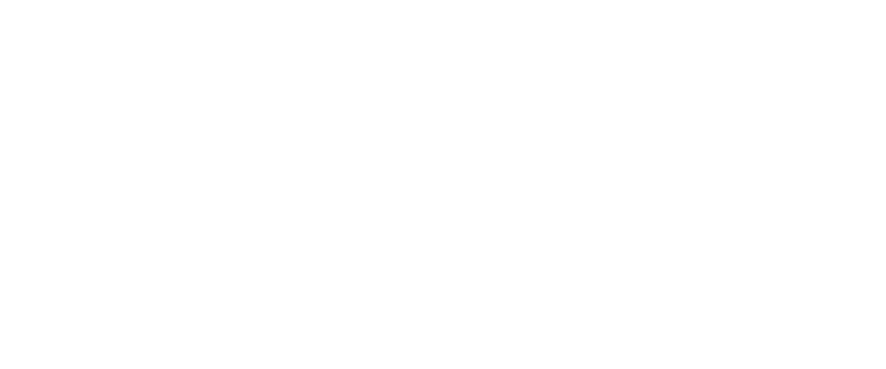## The equality of sets## Two sets are called equal if they are made from the same elements. With other words every element of one set is an element of the other set and conversely.

Example: The set A = {123456} and the set B = {123456} are equal because they have the same elements regardless the order of the elements.

## The subset of a set

The set B is called a subset of the set A if every element of the set B is included on the set A. In short we write B $\displaystyle \subset$ A

Example: Let’s have the set A = {123456} and the set B = {135}

We see that every element of the set B is an element of the set A. So we write B $\displaystyle \subset$ A.

{135} $\displaystyle \subset$ A = {123456}

## Type 1: Empty set

An empty set is a set which does not contain any element. It is written with the symbol Ø

Example: N = the set of all the natural numbers between -1 and 0.
As u can see there is no natural number between -1 and 0  In other words our set is an empty set N = Ø

Type 2: Finite set

A finite set is a set which has a finite number of elements and u can list them even when they are a lot.

## Example: E = the set of all the vowels of the alphabet

A = {123456}

A = {x: x $\displaystyle \in$ N, 2 < x < 10 }

## An infinite set is a set which has an infinite number of elements that can’t be listed even if we want to.

Example: N = the set of all the natural numbers

E = the set of all the even numbers

A = {x: x $\displaystyle \in$ Q, x > 2 } Q-rational number

Type 4: Universal set

Is a set that contains all the possible elements that you would consider for a set in a particular problem. The symbol used is U.

## What is the complement of a set?

The complement of a set A is the set of all things that are in U but not in the set A. The symbol A´ is used to denote the complement of a set A.

For example: If U={1,2,3,4,5,6,7,8,9,10}  and A={2,4,6}, then the complement of A would be A´= {1,3,5,7,8,9,10}

## Intersection of sets

Intersection of two sets is a set that contains all the common elements of two sets.
The symbol we use to write the intersection of two sets is $\displaystyle \cap$
The intersection of two sets A and B $\displaystyle \to A\cap B$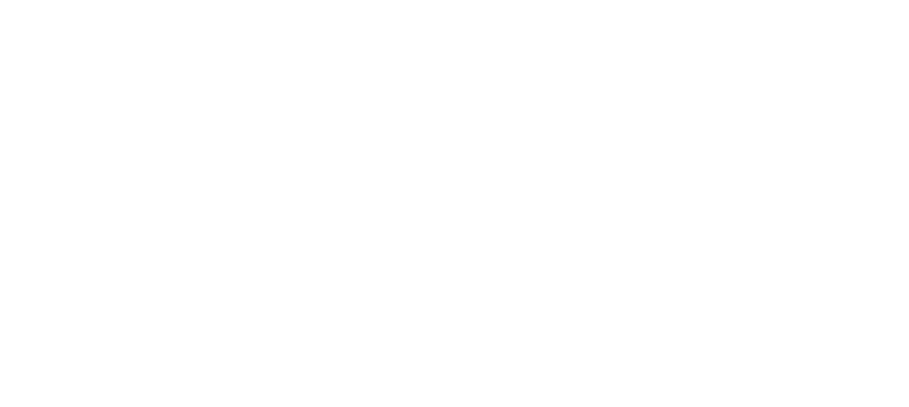$\displaystyle A\cap B=\left\{ x|\left( a\in A \right)\wedge \left( b\in B \right) \right\}$

### Union of the sets

Union of two sets is a set that contains all the elements of both of the sets.
The symbol we use to write the union of two sets is $\displaystyle \cup$.
The union of two sets A and B $\displaystyle \to A\cup B$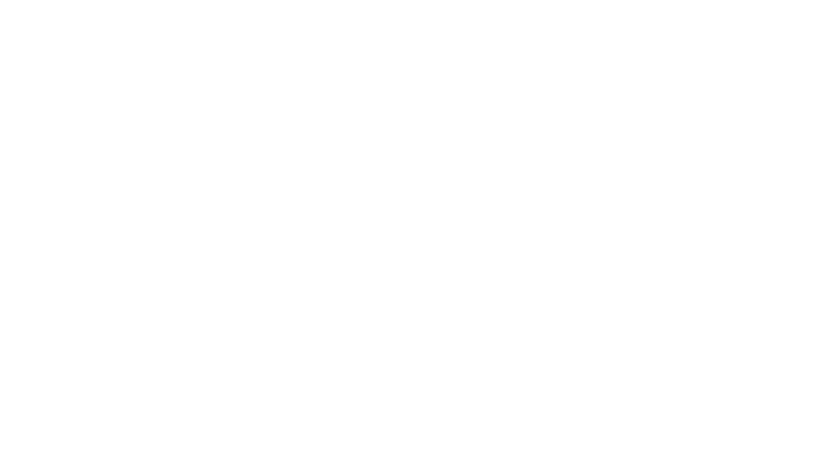$\displaystyle A\cup B=\left\{ x|\left( a\in A \right)\vee \left( b\in B \right) \right\}$

Example 1: Find the union and the intersection of the given sets.

A = {123456, 78}

B = {2481012}

Solution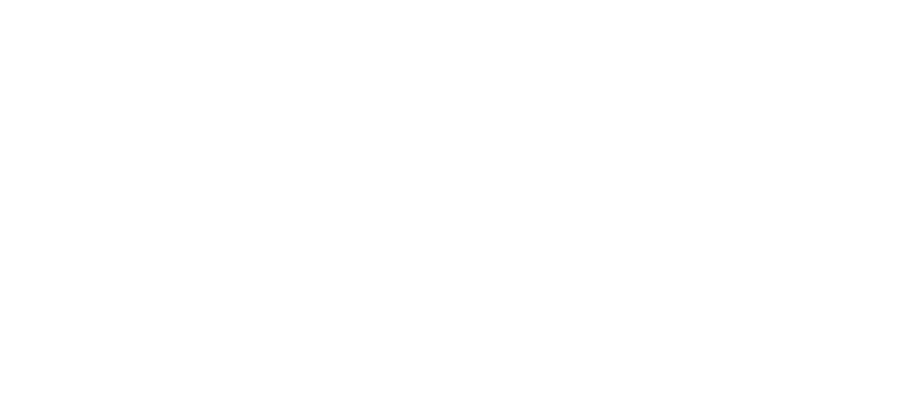The intersection of these two sets is:
A $\displaystyle \cap$ B = {2, 4, 8,}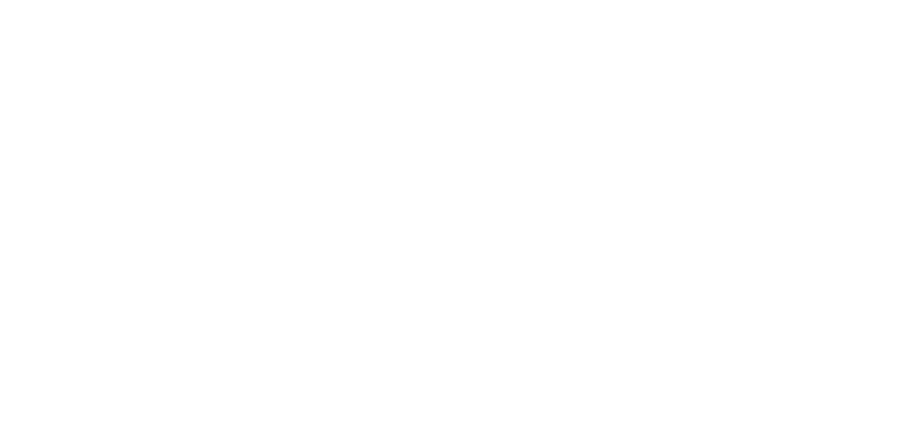## The union of these two sets is:

A $\displaystyle \cup$ B = {123456781012}

Example 2: If C= {4,8,12,16,20,24}  and D={5,8,20,24,28}

a) Lists the sets C∪D and C∩D.

b) Is it true that D⊂C ?

Solution

a) C∪D is the set of all members of C or of D or of both

C∪D= {4, 5, 8, 12, 16, 20, 24, 28}

C∩D is set of all elements that apper in both C and D

C∩D={8,20,24}

b)  Notice that 5∈D but 5∉C. So it is not true that every member of  D is also a member of C. So, D is not a subset of C.

Example 3: We have $\displaystyle A\cap B=\left\{ {a,b,c} \right\}$ and $\displaystyle A\cap C=\left\{ {a,b,c,d,e} \right\}$. Find how many elements has the set $\displaystyle A\cap (B\cup C)$?

Solution

$\displaystyle A\cap (B\cup C)=$$\displaystyle (A\cap B)\cup (A\cap C)= \displaystyle \left\{ {a,b,c} \right\}\cup \left\{ {a,b,c,d,e} \right\}=$$ \displaystyle \left\{ {a,b,c,d,e} \right\}$

$\displaystyle n\left\{ {A\cap (B\cup C)} \right\}=5$

Example 4: We have the number of elements on the set $\displaystyle n(A)=12$, $\displaystyle n(B)=10$ and $\displaystyle n(A\cup B)=18$. Find the number of elements $\displaystyle n(A/B)=?$

Solution

$\displaystyle n(A\cup B)=n(A)+n(B)-n(A\cap B)$

$\displaystyle n(A\cap B)=n(A)+n(B)-n(A\cup B)$

$\displaystyle n(A\cap B)=12+10-18=4$

$\displaystyle n(A/B)=n(A)-n(A\cap B)$

$\displaystyle n(A/B)=12-4=8$In summary:

• U represents a universal set

• represents the complement of set A

• is the symbol for union

• is the symbol for intersection

• BA indicates that B is a proper subset of A

• B A indicates that B is a subset of A but also equal to A. It is not a proper subset of A.

• BA indicates that B is not a proper subset of A.

• BA indicates that B is not a subset of A.